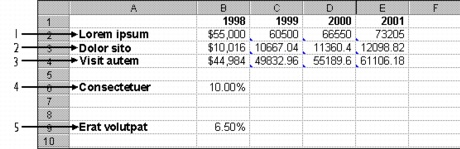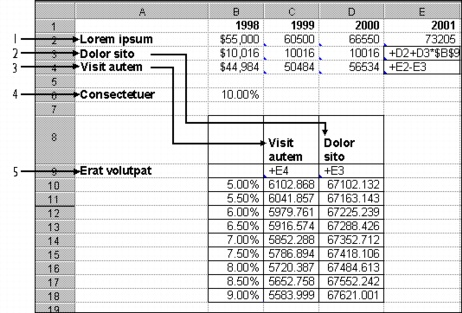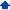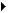### Creating one-variable What-If tables

What-If tables show the results of substituting a number of values for one or two variables in a formula. Also called sensitivity tables, What-If tables can answer questions such as “What if my company’s expenses increase 10%, 20%, 30%? And, what if production increases 10% to 25% at the same time?” What-If tables can also be used to look up values, such as postal charges for different parcel weights.

You can create one-variable What-If by varying a single value that is referenced by one or more What-If formulas. You can create one by setting up a column of figures to use as substitutions, and setting up formulas that reference a blank cell (the input cell). Each formula is calculated using the values in the substitution column, and the column below each formula is filled in with calculated values.

For example, suppose you want to set up a table that shows commissions earned for a range of sales amounts. By varying a single value referenced by a What-If formula, you can calculate the commission employees would receive for three different commission rates: 12%, 15%, and 18%. You can create a column of figures that reflect the sales range you want to show, and enter the formulas to calculate the commissions. Each formula must reference a blank cell. This is the input cell; each substitution value will be put in this cell, one at a time, to create the table. The input cell can be any cell outside the table.

To fill in the table values, Quattro Pro moves down each column, enters each row’s substitution value in the input cell, recalculates the formula at the top of the column, and places the result in the cell.

##### Using a one-variable table with a notebook database

A What-If table can be a helpful supplement to existing database information. You can create a table that uses information in a database without affecting the database itself. For information about creating a notebook database, see “Using databases.”

For example, you can determine what your net income might be in the year 2001. Suppose your boss promised you an annual raise of 10%, and the current inflation rate is 6.5%. You created a database projecting yearly gross income (based on a yearly 10% increase), expenses (based on a yearly 6.5% increase), and net income (subtracting expenses from gross income).A database showing one possibility (using one variable). 1) Gross Income 2) Business Expenses 3) Net Income 4) Salary Increase 5) Salary Increase.The same database using a What-If table to show the income based on a range of inflation rates. Cell B9 is the Input Cell. 1) Gross Income 2) Business Expenses 3) Net Income 4) Salary Increase 5) Inflation Rate.

#### To create a one-variable What-If table1 Type a column of values to substitute in one or more formulas.
 This will be the left column of the table.
 2 Type one or more formulas in the row above the first substitution value, starting in the column to the right.
 3 Click ToolsNumeric toolsWhat-If tables.
 4 Enable the One free variable option.
 5 Click the Range pickerin the What-If table box, and select the cells containing both formulas and substitution values.
 6 Click the Range pickerin the Input cell box, and select the blank cell referenced in the formulas.
 7 Click Generate.

#### To use a one-variable table with a notebook database1 Below a notebook database, type a range of data.
 2 Above and to the right of the list, enter the address of the cell that shows the formula which references the input cell.
 3 Move right one cell, then enter the cell address where you want to display the results.
 4 Click ToolsNumeric toolsWhat-If tables.
 5 Enable the One free variable option.
 6 Click the Range pickerin the What-If table box, and select the cells containing both formulas and substitution values.
 7 Click the Range pickerin the Input cell box, and select the blank cell referenced in the formulas.
 8 Click Generate.